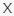# Find My Wrong Shape VideoQuizHero

Q 1. Take a look at our first cute critter! Can you find the shape that’s wrong?Choices:

A
B
C
D

A

Q 2. Which shape is wrong?Choices:

A
B
C
D

D

Q 3. Try not to get distracted by this lovable mouse! Can you spot the wrong shape?Choices:

A
B
C
D

A

Q 4. How about here? Can you spot the shape that’s wrong?Choices:

A
B
C
D

B

Q 5. Which shape is the odd one out?Choices:

A
B
C
D

B

Q 6. Which shape is different here?Choices:

A
B
C
D

C

Q 7. Can you identify the wrong shape?Choices:

A
B
C
D

D

Q 8. Think you can spot the different one?Choices:

A
B
C
D

A

Q 9. See if you can spot the different shape!Choices:

A
B
C
D

C

Q 10. Take a look! Which shape is wrong?Choices:

A
B
C
D

B

Q 11. Can you see the wrong shape?Choices:

A
B
C
D

B

Q 12. Which shape is the wrong one?Choices:

A
B
C
D

D

Q 13. Look closely! Can you find the wrong shape?Choices:A                                                   BC                                                    DE                                                     FE

Q 14. See if you can spot the wrong shape here!Choices:A                                                    BC                                                     DE                                                      FC

Q 15. Last one! Which shape is the wrong one?Choices:A                                                     BC                                                      DE                                                       F